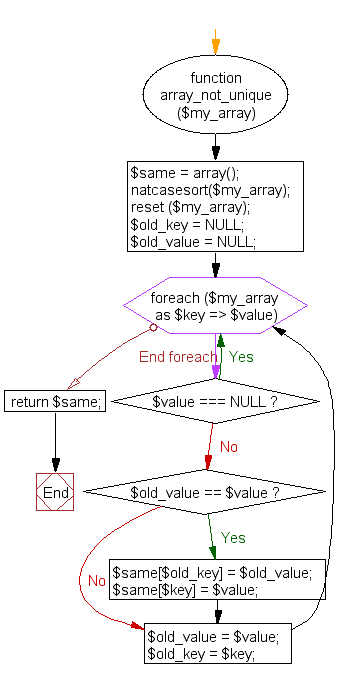﻿ PHP Array Exercise: Identify the email addresses which are not unique - w3resource# PHP Array Exercises : Identify the email addresses which are not unique

## PHP Array: Exercise-41 with Solution

Write a PHP program to identify the email addresses which are not unique.

Sample Solution:

PHP Code:

``````<?php
function array_not_unique(\$my_array) {
\$same = array();
natcasesort(\$my_array);
reset (\$my_array);

\$old_key    = NULL;
\$old_value    = NULL;
foreach (\$my_array as \$key => \$value) {
if (\$value === NULL) { continue; }
if (\$old_value == \$value) {
\$same[\$old_key]    = \$old_value;
\$same[\$key]        = \$value;
}
\$old_value    = \$value;
\$old_key    = \$key;
}
return \$same;
}

\$test_array = array();
\$test_array    = '[email protected]';
\$test_array    = '[email protected]';
\$test_array    = '[email protected]';
\$test_array    = '[email protected]';

print_r(array_not_unique(\$test_array));
?>
```
```

Sample Output:

```Array
(
 => [email protected]
 => [email protected]xample.com
)
```

Flowchart:PHP Code Editor:

What is the difficulty level of this exercise?

﻿

## PHP: Tips of the Day

PHP: Extract numbers from a string

```\$str = 'In My Cart : 11 12 items';
preg_match_all('!\d+!', \$str, \$matches);
print_r(\$matches);
```